Question

o reduce the drag coefficient and thus to improve the fuel efficiency, the frontal area of a car is to be reduced. Determine the amount of fuel and money saved per year as a result of reducing the frontal area from 18 to 14 ft2. Assume the car is driven 12,000 mi a year at an average speed of 55 mi/h. Take the density and price of gasoline to be 50 lbm/ft3 and $3.10/gal, respectively; the density of air to be 0.075 lbm/ft3, the heating value of gasoline to be 20,000 Btu/lbm; and the overall efficiency of the engine to be 30 percent. Take the drag coefficient as CD in progress 0 3 weeks 2021-08-30T02:06:07+00:00 1 Answers 0 views 0 ## Answers ( ) 1. Complete question To reduce the drag coefficient and thus to improve the fuel efficiency, the frontal area of a car is to be reduced. Determine the amount of fuel and money saved per year as a result of reducing the frontal area from 18 to 14 ft2. Assume the car is driven 12,000 mi a year at an average speed of 55 mi/h. Take the density and price of gasoline to be 50 lbm/ft3 and$3.10/gal, respectively; the density of air to be 0.075 lbm/ft3, the heating value of gasoline to be 20,000 Btu/lbm; and the overall efficiency of the engine to be 30 percent. Take the drag coefficient as CD=0.3 for a passenger car.

$57 Explanation: From the question we are told that Initial area of frontal area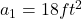Final area of frontal area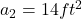Distance covered a year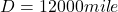Average speed a year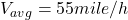Density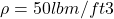Price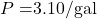Density of air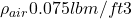Heating value of gasoline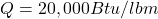Efficiency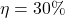Drag coefficient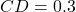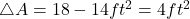Generally the equation for drag force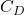is mathematically given as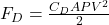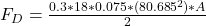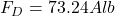where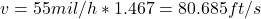Generally the equation for work done W is mathematically given as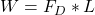where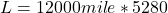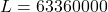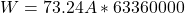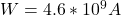Generally the equation for overall efficiency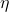is mathematically given as where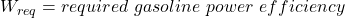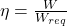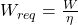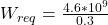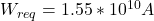Generally the equation for reduction fee with change in frontal area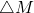is mathematically given as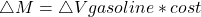Where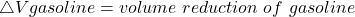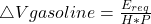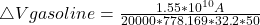if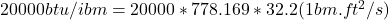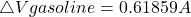Therefore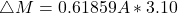if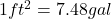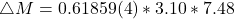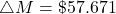Generally the equation for reduction of fuel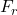is mathematically given as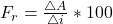where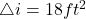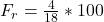Fuel reduction price by reducing front area is 22.22% Money saved per year is$57